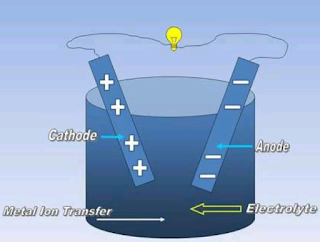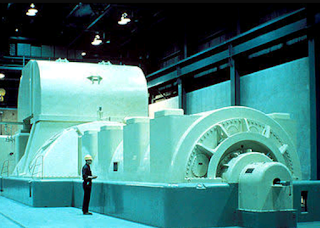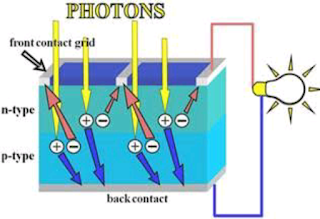# How We Can Transfer Or Convert Energy One Form To Another Form!!

## Electrical Energy Conversion

Another conceptual scheme upon which our thinking is based is the Conservation
Of Electrical Energy Or Electrical Energy Transfer.

By our training in the methods of science, we immediately becomes suspicious of any scheme that claim to create energy.

Here What Is The Relations Between Electrical Charge And Current : Electrical Charge And Electrical Current

The law of Conservation Of Energy states that energy can neither be created nor destroyed.

However  its form can be Converted One Fo To Another Form  i.e.  in Electrical Energy Conversion the Energy can be converted from one form of energy to another.

Electric Energy is the Energy Converted from some other form. There are a number ways in which this is accomplished.

Some of them are as follows:

(1)Electrochemical Energy  ConversionElectrochemical Energy Conversion

Electric batteries produce Electric Energy by the Conversion Of Chemical Energy.

A potentially important use of such batteries in the electric car Fuel cells are in this general classification.

(2)  Electromechanical Energy ConversionElectromechanical Energy Conversion

The Rotating Generator produces electric energy form Mechanical Energy Of Rotation.

Recommended Article Of Generators  :

Usually, the Mechanical energy is converted from thermal energy by a turbine and in turn, the thermal energy is converted from chemical energy by burning fossil fuel or form Nuclear fuel In Nuclear Power Plant .

Here How We Generate Electricity In The
Coal Based Thermal Power Station
Hydro Power Station
Nuclear Power Station

Sometimes, the conversion is from hydraulic energy by Hydroelectric Generation.

(3) Magneto hydro dynamics(MHD) energy conversionMagneto Hydro Dynamics Energy Conversion

Principal Of MHD Power Generation Is Work On Faraday's Law Of Electromagnetic Induction [ Thumb Rule For Magnetic Field ] .

These devise generated electric energy from the mechanical energy of a Hugh Velocity Ionised Gas.

(4) Photovoltaic Energy ConversionPhotovoltaic Energy Conversion

A class of devise are able to convert light energy directly in to electric energy.

The best Kon devise of this type is the Solar Cell.

(5) Thermo-Electric Energy ConversionThermo Electric Energy Conversion

When two dissimilar metal say copper and iron are joined together at two ends and a temperature different is maintained between the joints an Electric Current flows between the junctions.

• All these devise may be called energy source.

The function of each of these different sources of electric energy is the same in term of energy and charge.

In one form battery, for example, two metallic electrodes - one of zinc and one of copper are immersed in dilute sulphuric acid.

1.